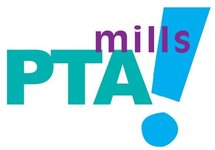## WANT TO BUY A YEARBOOK?

 /* styles */ Yearbooks are on sale in the front office for \$30 starting Monday 16th. -Limited quantities available. -First come first serve. -Cash or check -Make checks payable to Mills PTA and notate it is for a yearbook
 table div table+table+table+table div table{width:100%;padding:0}table div table+table+table+table div table img{width:96.23%;padding:0;float:none}table div table+table+table+table div table td{width:100%;padding:0 1.88% 18px}/* styles */## Track and Field Days - May 18th & 20th

Mills Track and Field Dates and Times
Wednesday May 18th, 2022
ESCE, PreK, and Kinder - 8:30am - 10:30am

Friday, May 20, 2022
3rd, 4th and 5th Grades 8:30 - 11:30

All students will be participating in rotation stations
Parents are welcome to attend

# Track and Field Volunteers Needed

Wanna have some fun in the sun while ​helping our Mountain Lions? Come out and volunteer for track and field.

Parent Volunteers are needed to help describe, model, and run stations for 3rd, 4th and 5th graders. Stations are set up all around the Mills Campus. Instructions for each station will be provided.
Volunteers needed on Friday, May 20th from 8:30am 11:40am. You can sign up for 1 hour or more.
Thank you,
Coach Norris

https://www.signupgenius.com/go/5080C44A8AF2CA3F85-track

 table div table+table+table+table+table+table+table div table{width:100%;padding:0}table div table+table+table+table+table+table+table div table img{width:96.23%;padding:0;float:none}table div table+table+table+table+table+table+table div table td{width:100%;padding:0 1.88% 18px}/* styles */## Epic Fun - May 20th

 table div table+table+table+table+table+table+table+table+table div table,table.module-8{width:32.08%;float:left;padding:0}table div table+table+table+table+table+table+table+table+table div table a{border:0 none;text-decoration:none}table div table+table+table+table+table+table+table+table+table div table img{width:100%!important;border:0 none;text-decoration:none}table div table+table+table+table+table+table+table+table+table div table td{width:100%;padding:0 20px 20px 0}/* styles */ Meet other Mountain Lions at Epic Fun on May 20th to help support Mills!
 table div table+table+table+table+table+table+table+table+table+table div table{width:100%;padding:0}table div table+table+table+table+table+table+table+table+table+table div table img{width:96.23%;padding:0;float:none}table div table+table+table+table+table+table+table+table+table+table div table td{width:100%;padding:0 1.88% 18px}/* styles */## Donate to International Medical Corps

 table div table+table+table+table+table+table+table+table+table+table+table+table+table div table{width:100%;padding:0}table div table+table+table+table+table+table+table+table+table+table+table+table+table div table img{width:96.23%;padding:0;float:none}table div table+table+table+table+table+table+table+table+table+table+table+table+table div table td{width:100%;padding:0 1.88% 18px}/* styles */## Safety Committee Volunteers Needed!

 table.module-14{width:99.62%;padding:0}table div table+table+table+table+table+table+table+table+table+table+table+table+table+table+table div table{width:99.62%;float:none;margin-left:auto;margin-right:auto;padding:0}table div table+table+table+table+table+table+table+table+table+table+table+table+table+table+table div table a{border:0 none;text-decoration:none}table div table+table+table+table+table+table+table+table+table+table+table+table+table+table+table div table img{width:100%!important;border:0 none;text-decoration:none}table div table+table+table+table+table+table+table+table+table+table+table+table+table+table+table div table td{width:100%;padding:0}/* styles */
 /* styles */ Please email May Martin at maymmartin@yahoo.com if you're interested in volunteering.
 table div table+table+table+table+table+table+table+table+table+table+table+table+table+table+table+table+table div table{width:100%;padding:0}table div table+table+table+table+table+table+table+table+table+table+table+table+table+table+table+table+table div table img{width:96.23%;padding:0;float:none}table div table+table+table+table+table+table+table+table+table+table+table+table+table+table+table+table+table div table td{width:100%;padding:0 1.88% 18px}/* styles */## Austin ISD Recognizes Mental Health Awareness Month

 table div table+table+table+table+table+table+table+table+table+table+table+table+table+table+table+table+table+table+table div table,table.module-18{width:32.83%;float:right;padding:0}table div table+table+table+table+table+table+table+table+table+table+table+table+table+table+table+table+table+table+table div table a{border:0 none;text-decoration:none}table div table+table+table+table+table+table+table+table+table+table+table+table+table+table+table+table+table+table+table div table img{width:100%!important;border:0 none;text-decoration:none}table div table+table+table+table+table+table+table+table+table+table+table+table+table+table+table+table+table+table+table div table td{width:100%;padding:0 0 20px 20px}/* styles */ May is Mental Health Awareness Month! Please view the flyer with live links to learn more about the resources AISD has shared.
 table div table+table+table+table+table+table+table+table+table+table+table+table+table+table+table+table+table+table+table+table div table{width:100%;padding:0}table div table+table+table+table+table+table+table+table+table+table+table+table+table+table+table+table+table+table+table+table div table img{width:96.23%;padding:0;float:none}table div table+table+table+table+table+table+table+table+table+table+table+table+table+table+table+table+table+table+table+table div table td{width:100%;padding:0 1.88% 18px}/* styles */## Affiliate News

 table.module-21{width:55.47%;padding:0}table div table+table+table+table+table+table+table+table+table+table+table+table+table+table+table+table+table+table+table+table+table+table div table{width:55.47%;float:none;margin-left:auto;margin-right:auto;padding:0}table div table+table+table+table+table+table+table+table+table+table+table+table+table+table+table+table+table+table+table+table+table+table div table a{border:0 none;text-decoration:none}table div table+table+table+table+table+table+table+table+table+table+table+table+table+table+table+table+table+table+table+table+table+table div table img{width:100%!important;border:0 none;text-decoration:none}table div table+table+table+table+table+table+table+table+table+table+table+table+table+table+table+table+table+table+table+table+table+table div table td{width:100%;padding:0}/* styles */
 table div table+table+table+table+table+table+table+table+table+table+table+table+table+table+table+table+table+table+table+table+table+table+table+table div table{width:100%;padding:0}table div table+table+table+table+table+table+table+table+table+table+table+table+table+table+table+table+table+table+table+table+table+table+table+table div table img{width:96.23%;padding:0;float:none}table div table+table+table+table+table+table+table+table+table+table+table+table+table+table+table+table+table+table+table+table+table+table+table+table div table td{width:100%;padding:0 1.88% 18px}/* styles */## Need to get your message out?

 /* styles */ Submit info to the Mills Messenger. Want to see the Mills Calendar on your Google calendar? Add Mills Calendar to my Google Calendar. Have a friend who isn't getting the Messenger but wants to? Send them this link to sign up: https://madmimi.com/signups/join/7059
 table div table+table+table+table+table+table+table+table+table+table+table+table+table+table+table+table+table+table+table+table+table+table+table+table+table+table+table div table{width:100%;padding:0}table div table+table+table+table+table+table+table+table+table+table+table+table+table+table+table+table+table+table+table+table+table+table+table+table+table+table+table div table img{width:96.23%;padding:0;float:none}table div table+table+table+table+table+table+table+table+table+table+table+table+table+table+table+table+table+table+table+table+table+table+table+table+table+table+table div table td{width:100%;padding:0 1.88% 18px}/* styles *//* styles */ Find recent editions of the Mills Messenger at millspta.org.